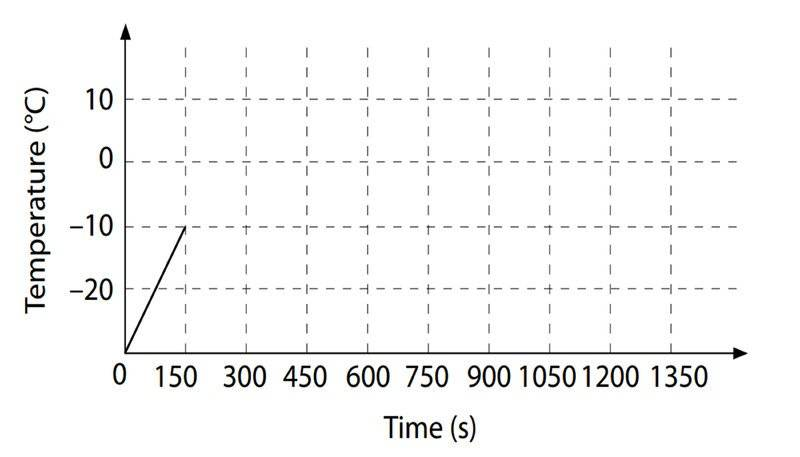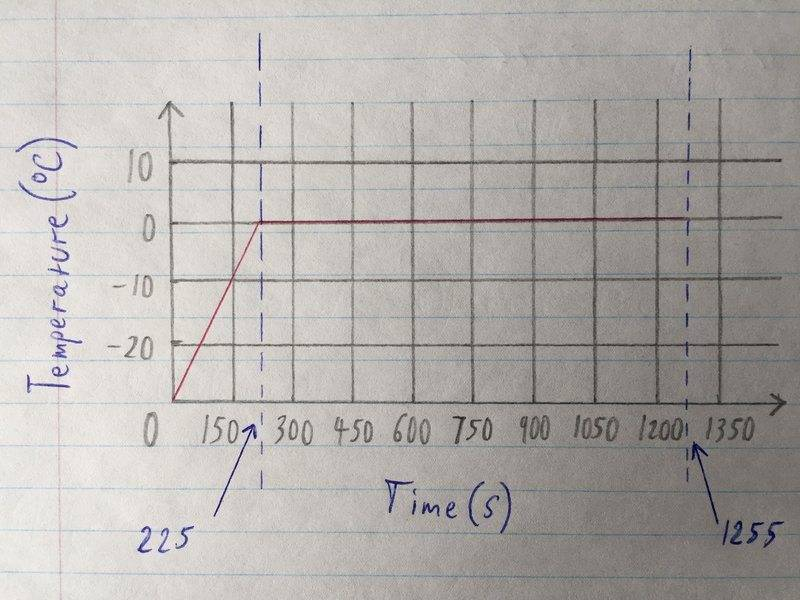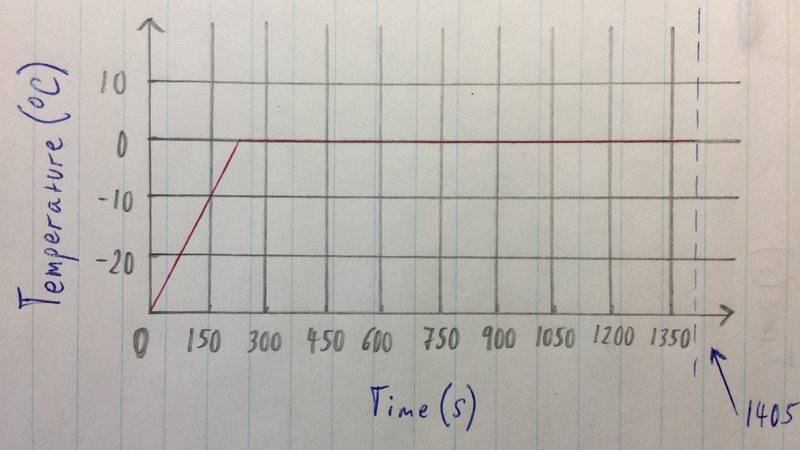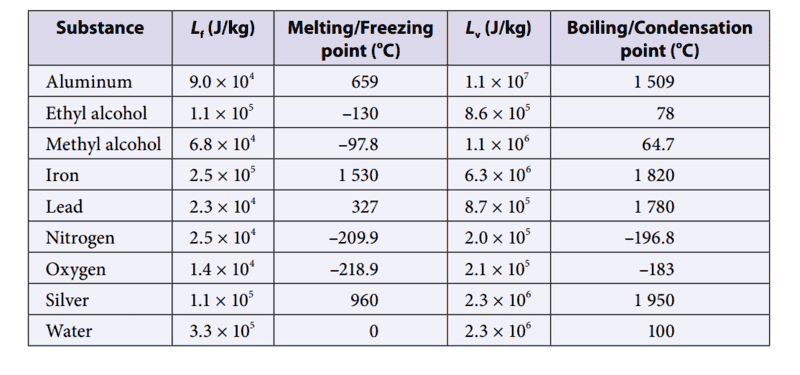# Heat transfer, how long it takes ice to reach melting point

## Homework Statement

A 0.25 kg piece of ice at -30 C is warmed by an electric heater and the following graph of temperature is produced. Assume that there has been no loss of energy to the surroundings.- Use the info on the graph to determine the power output of the heater
- Explain how long it will take to get the ice to the melting point of 0 C
- Explain how long it will take to melt completely once the ice reaches 0 C
- Create a graph like the one above for when the ice starts melting and then complete it, up to this point

Q = micecicetice
E=Q
P=Q/t
t=Q/P

## The Attempt at a Solution

First calculating the energy:
Q = mct = 0.25 kg x (2.1 x 103 J) x (-30 C - 10 C) = 10, 500 J
Then the power:
P = Q/t = 10, 500J / 150s = 70 W

Now to to find out how long it takes the ice to get to the melting point:
Q = mct = 0.25 kg x (2.1 x 103J) x (-10 C - 0 C) = 5250 J
t = Q/P = 5250 / 70 = 75s

How long it will take the ice to melt completely once it reaches 0 C:
Q = mLf = 0.25 kg x (3.3 x 105) = 82 500 J
t = Q/P = 82500 / 70 = 1180s approximately

Then, 1180s + 75s = 1255s
This is the approximate time it will take the ice to go from -10 C to 0 C, and then completely melt.

The graph:Have I done everything correctly? Any confirmation and/or help is greatly appreciated.

## Answers and Replies

haruspex
Science Advisor
Homework Helper
Gold Member
2020 Award
Have I done everything correctly?
I believe the latent heat of fusion of ice is more like 3.34x105Jkg-1. If you round it off to two sig figs then you need to quote fewer in your answer.

Don't you need to include the initial 150s in deciding the end point of the graph?

Other than that, looks fine.

•Humbleness
Don't you need to include the initial 150s in deciding the end point of the graph?

Makes sense, as the question stated from when the ice starts melting...
That would lead to 1255s + 150s = 1405sDoes this look better?

#### Attachments

haruspex
Science Advisor
Homework Helper
Gold Member
2020 Award
Makes sense, as the question stated from when the ice starts melting...
That would lead to 1255s + 150s = 1405s
View attachment 221005

Does this look better?
Yes, but as I posted you should either round it off to 1400s or use a more accurate value for the latent heat of fusion (which I think will make it nearly 1420s).

•Humbleness
Understood, even though I was following this table in my textbook:However, I have calculated again using your advice, and rounded up to exactly 1418s in my calculations. I don't think the teacher marking will expect me to know of 3.34 x 105
May I ask from where did you find that info? I could possibly cite the source and write it down with these new calculations, as a justification for my response.

#### Attachments

haruspex
Science Advisor
Homework Helper
Gold Member
2020 Award
Understood, even though I was following this table in my textbook:
View attachment 221006
However, I have calculated again using your advice, and rounded up to exactly 1418s in my calculations. I don't think the teacher marking will expect me to know of 3.34 x 105
May I ask from where did you find that info? I could possibly cite the source and write it down with these new calculations, as a justification for my response.
If you are given 3.3 then use that, but it is not given to you as 3.30, so you should only quote two sig figs in the answer: 1400s.

•Humbleness
Which is exactly what I ended up doing. Thank you so much for your help.